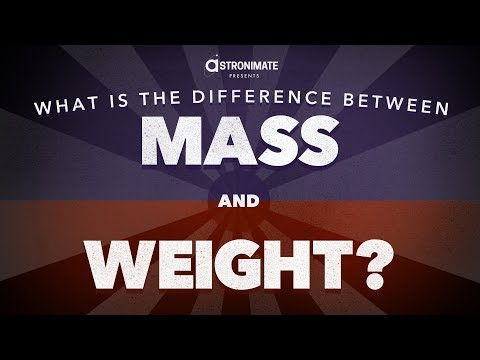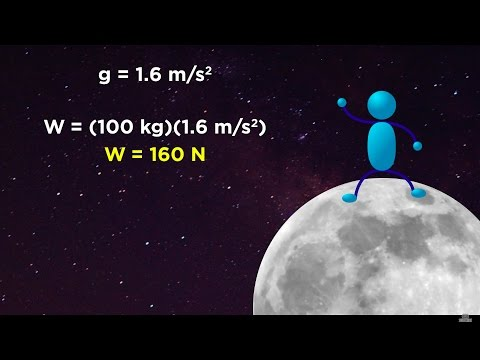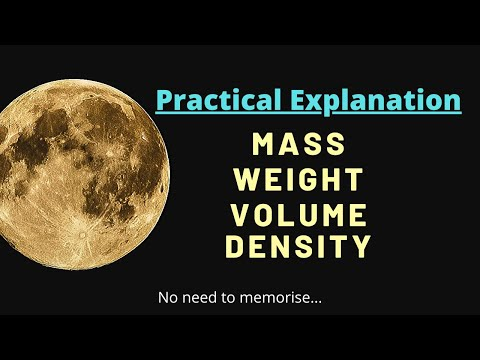## Mass and Weight

Subject: Science

#### Overview

The amount of matter contained in the body is called mass of the body. It is measured in kg. The weight of a body is defined as the force with which it is attracted towards the center of the planet. It is measured in Newton.

##### Mass and Weight

Mass

Mass of a body is defined by the amount of matter contained in that body. It remains the same in every part of the universe. We can use a physical balance or a beam balance to measure the mass of the body. For example: The equilibrium state of a beam balance with 2 kg standard mass in one pan and 2 kg of rice in another pan, the force of gravity in both pans are equal.

Hence, the mass of a body can be determined by comparing the force of gravity on the standard mass and the body to be weighted by using a physical balance or a beam balance. The unit of mass is ‘kg’ in the SI system. The mass of a body depends upon the number of atoms and the average mass of atoms present in the body. It is a scalar quantity.

Weight

Weight of a body is defined as the force with which a body is attracted towards the center of a planet. We can measure the weight of a body using a spring balance. The unit of weight is ‘Newton’ (N) in the SI system and ‘Dyne’ in the CGS system. Since weight depends upon the value of acceleration due to the gravity of planets (g), its value changes from place to place. It is a vector quantity.Mathematically,

W = m × g

Where, W = weight of a body

m = mass of a body

g = acceleration due to gravity

As the value of acceleration due to gravity (g) depends on the radius, depth, and height from the earth’s surface, weight is also affected by the same factors. The value of g is directly proportional to the weight of a body (i.e. w α g). So, the higher the value of g, higher will be the weight and lower the value of g, lower will be weight. For example: the weight of an object will be more in Terai than that at the top of Mount Everest.

Differences between Mass and Weight

The major differences are:

 Mass Weight 1. Mass is the amount of matter contained in a body. 1. Weight is the force with which a body is attracted towards the center of a planet. 2. Its value remains the same in every part of the universe. 2. Its value differs from place to place. 3. It is a scalar quantity. 3. It is a vector quantity. 4. It can be measured by using a physical balance or a beam balance. 4. It can be measured by using a spring balance. 5. It is measured in kilograms (kg). 5. It is measured in newton (N).

##### Things to remember
1. Mass of a body is the amount of matter contained in a body.
2. Mass is the scalar quantity.
3. Mass is measured in kilograms (kg) in the SI system.
4. Weight of a body is the force with which it is attracted towards the center of the planet.
5. Weight depends upon the value of acceleration due to the gravity of planets.
6. The value of g is maximum at the poles, so weight at the poles is more.
7. The value of g is minimum at the equator, so weight at the equator is less.
8. The value of g decreases with the increase in altitude and depth, so the weight also decreases with the increment in altitude and depth.
9. The value of g is zero at the center of the earth, so the weight of a body at the center of the earth is also zero.
• It includes every relationship which established among the people.
• There can be more than one community in a society. Community smaller than society.
• It is a network of social relationships which cannot see or touched.
• common interests and common objectives are not necessary for society.
##### Videos for Mass and Weight##### Less Than Five - What’s the Difference Between Mass and Weight?##### Mass vs. Weight and the Normal Force##### Physics - Are Mass and Weight the same thing?##### What is mass, weight, volume and density?

The differences between mass and weight are mentioned as follows:

#### Weight

1.  The amount of matter contained in a body is called mass. 1. The force with which a body is attracted to the centre of a planet is called weight.
2. The value of mass is same everywhere in the universe. 2. The value of weight differs from place to place.
3. Mass is a scalar quantity. 3. Weight is a vector quantity.
4. Mass is measured in kilograms (kg). 4. Weight is measured in newton (N).
5. Mass is measured by using a physical balance. 5. Weight is measured by using a spring balance.

The amount of matter contained in a body is called the mass of that body. It is a scalar quantity. It remains the same everywhere in the universe. Its SI unit is kilogram (kg).

The weight of a body is defined as the force with which a body is attracted to the centre of a planet. It is a vector quantity. Its value differs from place to place. Its SI unit is newton (N).

The body has more weight at the poles as the value of g is maximum at the poles of the Earth, so the weight of the body is maximum at the poles.